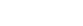# TSNI / TSNI-integral

:: DESCRIPTION

TSNI assumes that the gene network can be modeled by the following system of ordinary differential equation to represent the rate of synthesis of a transcript as a function of the concentrations of every other transcript in a cell and the external perturbation.

TSNI-integral

::DEVELOPER

di Bernardo Lab

:: SCREENSHOTS

N/A

:: REQUIREMENTS

• Linux / Windows / MacOsX
• MatLabTSNI / TSNI-integral